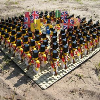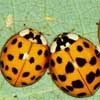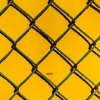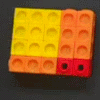# Resources tagged with: Expanding and factorising quadratics

Filter by: Content type:
Age range:
Challenge level:

### There are 35 results

Broad Topics > Algebraic expressions, equations and formulae > Expanding and factorising quadratics### Always Two

##### Age 14 to 18Challenge Level

Find all the triples of numbers a, b, c such that each one of them plus the product of the other two is always 2.### Parabella

##### Age 16 to 18Challenge Level

This is a beautiful result involving a parabola and parallels.### Poly Fibs

##### Age 16 to 18Challenge Level

A sequence of polynomials starts 0, 1 and each poly is given by combining the two polys in the sequence just before it. Investigate and prove results about the roots of the polys.### Telescoping Functions

##### Age 16 to 18

Take a complicated fraction with the product of five quartics top and bottom and reduce this to a whole number. This is a numerical example involving some clever algebra.### Always Perfect

##### Age 14 to 18Challenge Level

Show that if you add 1 to the product of four consecutive numbers the answer is ALWAYS a perfect square.### Two Cubes

##### Age 14 to 16Challenge Level

Two cubes, each with integral side lengths, have a combined volume equal to the total of the lengths of their edges. How big are the cubes? [If you find a result by 'trial and error' you'll need to. . . .### Fibonacci Factors

##### Age 16 to 18Challenge Level

For which values of n is the Fibonacci number fn even? Which Fibonnaci numbers are divisible by 3?### Common Divisor

##### Age 14 to 16Challenge Level

Find the largest integer which divides every member of the following sequence: 1^5-1, 2^5-2, 3^5-3, ... n^5-n.### Unit Interval

##### Age 16 to 18 ShortChallenge Level

Can you prove our inequality holds for all values of x and y between 0 and 1?### Mega Quadratic Equations

##### Age 16 to 18Challenge Level

What do you get when you raise a quadratic to the power of a quadratic?### Spinners

##### Age 16 to 18Challenge Level

How do scores on dice and factors of polynomials relate to each other?### Fair Shares?

##### Age 14 to 16Challenge Level

A mother wants to share a sum of money by giving each of her children in turn a lump sum plus a fraction of the remainder. How can she do this in order to share the money out equally?### Hollow Squares

##### Age 14 to 16Challenge Level

Which armies can be arranged in hollow square fighting formations?### Square Number Surprises

##### Age 14 to 16Challenge Level

There are unexpected discoveries to be made about square numbers...### Difference of Two Squares

##### Age 14 to 16Challenge Level

What is special about the difference between squares of numbers adjacent to multiples of three?### Spot the Difference

##### Age 16 to 18 ShortChallenge Level

If you plot these graphs they may look the same, but are they?### What's Possible?

##### Age 14 to 16Challenge Level

Many numbers can be expressed as the difference of two perfect squares. What do you notice about the numbers you CANNOT make?### Finding Factors

##### Age 14 to 16Challenge Level

Can you find the hidden factors which multiply together to produce each quadratic expression?### Factorising with Multilink

##### Age 14 to 16Challenge Level

Can you find out what is special about the dimensions of rectangles you can make with squares, sticks and units?### Pair Products

##### Age 14 to 16Challenge Level

Choose four consecutive whole numbers. Multiply the first and last numbers together. Multiply the middle pair together. What do you notice?### Geometric Parabola

##### Age 14 to 16Challenge Level

Explore what happens when you draw graphs of quadratic equations with coefficients based on a geometric sequence.### Never Prime

##### Age 14 to 16Challenge Level

If a two digit number has its digits reversed and the smaller of the two numbers is subtracted from the larger, prove the difference can never be prime.### Polar Flower

##### Age 16 to 18Challenge Level

This polar equation is a quadratic. Plot the graph given by each factor to draw the flower.### Perfectly Square

##### Age 14 to 16Challenge Level

The sums of the squares of three related numbers is also a perfect square - can you explain why?### Number Rules - OK

##### Age 14 to 16Challenge Level

Can you produce convincing arguments that a selection of statements about numbers are true?### 2-digit Square

##### Age 14 to 16Challenge Level

A 2-Digit number is squared. When this 2-digit number is reversed and squared, the difference between the squares is also a square. What is the 2-digit number?### Why 24?

##### Age 14 to 16Challenge Level

Take any prime number greater than 3 , square it and subtract one. Working on the building blocks will help you to explain what is special about your results.### Code to Zero

##### Age 16 to 18Challenge Level

Find all 3 digit numbers such that by adding the first digit, the square of the second and the cube of the third you get the original number, for example 1 + 3^2 + 5^3 = 135.### Multiplication Magic

##### Age 14 to 16Challenge Level

Given any 3 digit number you can use the given digits and name another number which is divisible by 37 (e.g. given 628 you say 628371 is divisible by 37 because you know that 6+3 = 2+7 = 8+1 = 9). . . .### Composite Notions

##### Age 14 to 16Challenge Level

A composite number is one that is neither prime nor 1. Show that 10201 is composite in any base.### Pythagoras Perimeters

##### Age 14 to 16Challenge Level

If you know the perimeter of a right angled triangle, what can you say about the area?### Iff

##### Age 14 to 18Challenge Level

Take a triangular number, multiply it by 8 and add 1. What is special about your answer? Can you prove it?### Plus Minus

##### Age 14 to 16Challenge Level

Can you explain the surprising results Jo found when she calculated the difference between square numbers?### Novemberish

##### Age 14 to 16Challenge Level

a) A four digit number (in base 10) aabb is a perfect square. Discuss ways of systematically finding this number. (b) Prove that 11^{10}-1 is divisible by 100.### Powerful Factors

##### Age 16 to 18Challenge Level

Use the fact that: x²-y² = (x-y)(x+y) and x³+y³ = (x+y) (x²-xy+y²) to find the highest power of 2 and the highest power of 3 which divide 5^{36}-1.